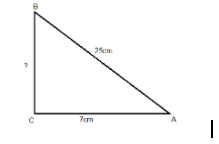Courses
Courses for Kids
Free study material
Free LIVE classes
MoreLIVE
Join Vedantu’s FREE Mastercalss

# ABC is a triangle, right angled at $C$. If $AB = 25cm$ and $AC = 7cm$, find BC.Verified
365.4k+ views
Hint: Draw the diagram of the right triangle to find out which of the three sides is
the hypotenuse, base or perpendicular. Using Pythagoras theorem, find the unknown side.We are given that the $ABC$ is a triangle, right angled at $C$
Then, $AB$ is the hypotenuse,$AC$ is the base and $BC$ perpendicular
Now, according to the Pythagoras theorem we know that,
${({\text{hypotenuse}})^2} = {(base)^2} + {(perpendicular)^2}$
Therefore, using this we get,
${({\text{AB}})^2} = {(AC)^2} + {(BC)^2}$
Now after substituting the given values we get,
$\Rightarrow {({\text{7}})^2} = {(25)^2} + {(BC)^2}$
$\Rightarrow {(BC)^2} = {(25)^2} - {({\text{7}})^2}$
$\Rightarrow {(BC)^2} = 625 - 49$
$\Rightarrow {(BC)^2} = 576$
$\Rightarrow BC = 24$
$\therefore BC = 24cm$
So, this is the required solution.

Note: In order to solve these types of questions, simply put the given values of the sides of
the right triangle in the Pythagoras theorem and evaluate it to obtain the required solution.

Last updated date: 29th Sep 2023
Total views: 365.4k
Views today: 9.65k